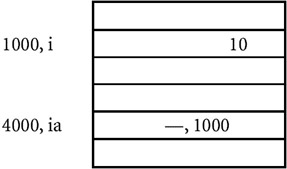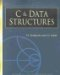### Introduction

For every variable declared in a program there is some memory allocation. Memory is specified in arrays of bytes, the size of which depending on the type of variable. For the integer type, 2 bytes are allocated, for floats, 4 bytes are allocated, etc. For every variable there are two attributes: address and value, described as follows:

Program

```#include
main ()
{
int i, j, k; //A
i = 10; //B
j = 20; //C
k = i + j; //D

printf ("Value of k is %d
", k);
}
```

Explanation

1. Memory allocations to the variables can be explained using the following variables:

```100,i 10
200, j 20
300,k 30
```

When you declare variables i, j, k, memory is allocated for storing the values of the variables. For example, 2 bytes are allocated for i, at location 100, 2 bytes are allocated for j at location 200, and 2 bytes allocated for k at location 300. Here 100 is called the address of i, 200 is called address of j, and 300 is called the address of k.

2. When you execute the statement i = 10, the value 10 is written at location 100, which is specified in the figure. Now, the address of i is 100 and the value is 10. During the lifetime of variables, the address will remain fixed and the value may be changed. Similarly, value 20 is written at address 200 for j.
3. During execution, addresses of the variables are taken according to the type of variable, that is, local or global. Local variables usually have allocation in stack while global variables are stored in runtime storage.

Points to Remember

1. Each variable has two attributes: address and value.
2. The address is the location in memory where the value of the variable is stored.
3. During the lifetime of the variable, the address is not changed but the value may change.

## POINTERS

### Introduction

A pointer is a variable whose value is also an address. As described earlier, each variable has two attributes: address and value. A variable can take any value specified by its data type. For example, if the variable i is of the integer type, it can take any value permitted in the range specified by the integer data type. A pointer to an integer is a variable that can store the address of that integer.

Program

```#include
main ()
{
int i; //A
int * ia; //B
i = 10; //C
ia = &i; //D

printf (" The address of i is %8u
", ia); //E
printf (" The value at that location is %d
", i); //F
printf (" The value at that location is %d
", *ia); //G
*ia = 50; //H
printf ("The value of i is %d
", i); //I
}
```

Explanation

1. The program declares two variables, so memory is allocated for two variables. i is of the type of int, and ia can store the address of an integer, so it is a pointer to an integer.
2. The memory allocation is as follows:4. When you execute i = 10, 10 is written at location 1000.
5. When you execute ia = &i then the address and value are assigned to i, thus i has the address of 4000 and value is 1000.
6. You can print the value of i by using the format %au because addresses are usually in the format unsigned long, as given in statement E.
7. Statement F prints the value of i, (at the location 1000).
8. Alternatively, you can print the value at location 1000 using statement G. *ia means you are printing the value at the location specified by ia. Since i has the value for 1000, it will print the value at location 1000.
9. When you execute *ia = 50, which is specified by statement H, the value 50 is written at the location by ia. Since ia specifies the location 1000, the value at the location 1000 is written as 50.
10. Since i also has the location 1000, the value of i gets changed automatically from 10 to 50, which is confirmed from the printf statement written at position i.

Points to Remember

1. Pointers give a facility to access the value of a variable indirectly.
2. You can define a pointer by including a* before the name of the variable.
3. You can get the address where a variable is stored by using &.C & Data Structures (Charles River Media Computer Engineering)
ISBN: 1584503386
EAN: 2147483647
Year: 2006
Pages: 232